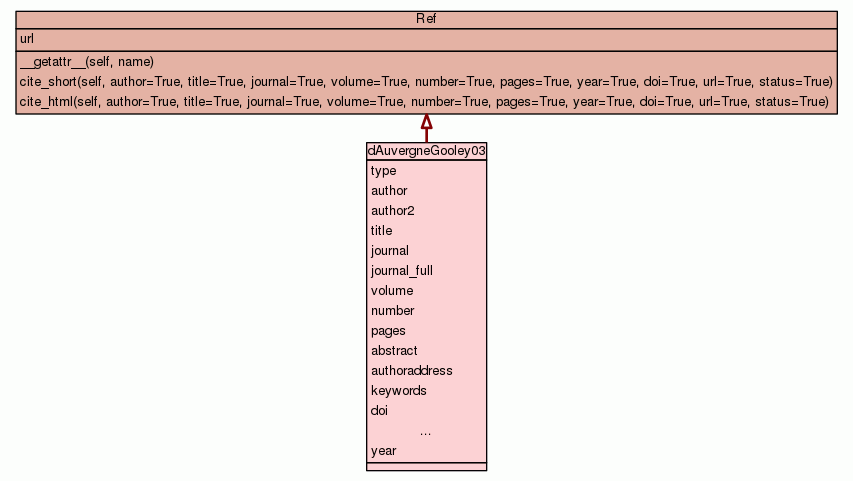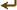[frames] | no frames]

# Class dAuvergneGooley03

source codeBibliography container.

 Instance Methods

Inherited from `Ref`: `__getattr__`, `cite_html`, `cite_short`

 Class Variables
type = `'journal'`
hash(x)
author = `'d\'Auvergne, E. J. and Gooley, P. R.'`
hash(x)
author2 = `[['Edward', 'd\'Auvergne', 'E.', 'J.'], ['Paul', 'Go...`
hash(x)
title = `'The use of model selection in the model-free analysis...`
hash(x)
journal = `'J. Biomol. NMR'`
hash(x)
journal_full = `'Journal of Biomolecular NMR'`
hash(x)
volume = `'25'`
hash(x)
number = `'1'`
hash(x)
pages = `'25-39'`
hash(x)
abstract = `'Model-free analysis of NMR relaxation data, which ...`
authoraddress = `'Department of Biochemistry and Molecular Biol...`
keywords = `'Amines ; Diffusion ; *Models, Molecular ; Motion ;...`
doi = `'10.1023/A:1021902006114'`
hash(x)
pubmed_id = `12566997`
hash(x)
status = `'published'`
hash(x)
year = `2003`
hash(x)

Inherited from `Ref`: `url`

 Class Variable Details

### author2

hash(x)

Value:
 ````[``[``'``Edward``'``, ``'``d\'Auvergne``'``, ``'``E.``'``, ``'``J.``'``]``,` `[``'``Paul``'``, ``'``Gooley``'``, ``'``P.``'``, ``'``R.``'``]``]` ```

### title

hash(x)

Value:
 ````'``The use of model selection in the model-free analysis of protein dyna``mics.``'` ```

### abstract

Value:
 ````'``Model-free analysis of NMR relaxation data, which is widely used for ``the study of protein dynamics, consists of the separation of the globa``l rotational diffusion from internal motions relative to the diffusion`` frame and the description of these internal motions by amplitude and ``timescale. Five model-free models exist, each of which describes a dif``ferent type of motion. Model-free analysis requires the selection of t``he model which best describes the dynamics of the NH bond. It will be ``demonstrated that the model selection technique currently used has two``...` ```

 ````'``Department of Biochemistry and Molecular Biology, University of Melbo``urne, Melbourne, Victoria 3010, Australia.``'` ```
 ````'``Amines ; Diffusion ; *Models, Molecular ; Motion ; Nuclear Magnetic R``esonance, Biomolecular/*methods ; Proteins/*chemistry ; Research Suppo``rt, Non-U.S. Gov\'t ; Rotation``'` ```